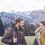# Proof Contest Day 2

For any regular $n$ sided polygon, if we choose any point inside the polygon (not on side) and drop one perpendicular to every side from the point prove that the sum of all the heights remain constant, irrespective where the points lies inside the polygon.Note by Department 8
5 years, 7 months ago

This discussion board is a place to discuss our Daily Challenges and the math and science related to those challenges. Explanations are more than just a solution — they should explain the steps and thinking strategies that you used to obtain the solution. Comments should further the discussion of math and science.

When posting on Brilliant:

• Use the emojis to react to an explanation, whether you're congratulating a job well done , or just really confused .
• Ask specific questions about the challenge or the steps in somebody's explanation. Well-posed questions can add a lot to the discussion, but posting "I don't understand!" doesn't help anyone.
• Try to contribute something new to the discussion, whether it is an extension, generalization or other idea related to the challenge.

MarkdownAppears as
*italics* or _italics_ italics
**bold** or __bold__ bold
- bulleted- list
• bulleted
• list
1. numbered2. list
1. numbered
2. list
Note: you must add a full line of space before and after lists for them to show up correctly
paragraph 1paragraph 2

paragraph 1

paragraph 2

[example link](https://brilliant.org)example link
> This is a quote
This is a quote
    # I indented these lines
# 4 spaces, and now they show
# up as a code block.

print "hello world"
# I indented these lines
# 4 spaces, and now they show
# up as a code block.

print "hello world"
MathAppears as
Remember to wrap math in $$ ... $$ or $ ... $ to ensure proper formatting.
2 \times 3 $2 \times 3$
2^{34} $2^{34}$
a_{i-1} $a_{i-1}$
\frac{2}{3} $\frac{2}{3}$
\sqrt{2} $\sqrt{2}$
\sum_{i=1}^3 $\sum_{i=1}^3$
\sin \theta $\sin \theta$
\boxed{123} $\boxed{123}$

Sort by:

Let the side of each polygon be $b$. And join the vertices with the point.

Now area of each triangle (formed by two adjacent vertices of the polygon and that point), will be $\frac12bh_i$ where $h_i$ is one of those heights.

Now, the sum of all the triangles would equal the area of the polygon, let the total area of polygon be $A$.

So we get $\frac12b(h_1+h_2+...+h_n)=A$ And thus,

$\sum h_i=\frac{2A}{b}$

This shows it will always be constant until the point is in the polygon.

- 5 years, 7 months ago

I have edited out your solution. Nice (+1)

- 5 years, 7 months ago

Oops !! I didn't saw your solution and you beated me while writing the solution.

- 5 years, 7 months ago

No problem brother. (y)

- 5 years, 7 months ago

You would have fun solving Follow Up Problem. :)

- 5 years, 6 months ago